JEE  >  Test: JEE Main 35 Year PYQs- Differentiation

# Test: JEE Main 35 Year PYQs- Differentiation - JEE

Test Description

## 16 Questions MCQ Test Mathematics (Maths) for JEE Main & Advanced - Test: JEE Main 35 Year PYQs- Differentiation

Test: JEE Main 35 Year PYQs- Differentiation for JEE 2023 is part of Mathematics (Maths) for JEE Main & Advanced preparation. The Test: JEE Main 35 Year PYQs- Differentiation questions and answers have been prepared according to the JEE exam syllabus.The Test: JEE Main 35 Year PYQs- Differentiation MCQs are made for JEE 2023 Exam. Find important definitions, questions, notes, meanings, examples, exercises, MCQs and online tests for Test: JEE Main 35 Year PYQs- Differentiation below.
Solutions of Test: JEE Main 35 Year PYQs- Differentiation questions in English are available as part of our Mathematics (Maths) for JEE Main & Advanced for JEE & Test: JEE Main 35 Year PYQs- Differentiation solutions in Hindi for Mathematics (Maths) for JEE Main & Advanced course. Download more important topics, notes, lectures and mock test series for JEE Exam by signing up for free. Attempt Test: JEE Main 35 Year PYQs- Differentiation | 16 questions in 30 minutes | Mock test for JEE preparation | Free important questions MCQ to study Mathematics (Maths) for JEE Main & Advanced for JEE Exam | Download free PDF with solutions
 1 Crore+ students have signed up on EduRev. Have you?
Test: JEE Main 35 Year PYQs- Differentiation - Question 1

###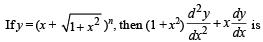Detailed Solution for Test: JEE Main 35 Year PYQs- Differentiation - Question 1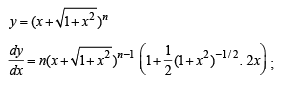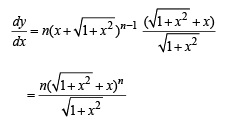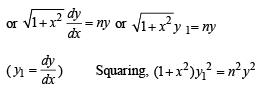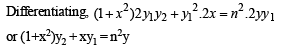Test: JEE Main 35 Year PYQs- Differentiation - Question 2

### If f ( y) = ey , g (y) = y; y > 0 and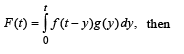Detailed Solution for Test: JEE Main 35 Year PYQs- Differentiation - Question 2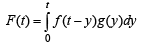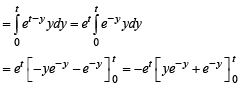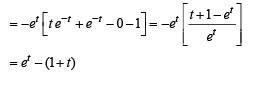Test: JEE Main 35 Year PYQs- Differentiation - Question 3

### If  f (x) = xn , then the value of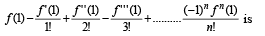Detailed Solution for Test: JEE Main 35 Year PYQs- Differentiation - Question 3

f (x) = xn ⇒ f (1) = 1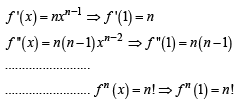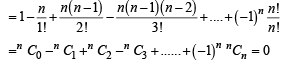Test: JEE Main 35 Year PYQs- Differentiation - Question 4

Let f(x) be a polynomial fun ction of second degree. If f(1) = f (– 1) and a, b, c are in A. P , then f '(a), f '(b), f '(c) are in

Detailed Solution for Test: JEE Main 35 Year PYQs- Differentiation - Question 4

f(x) = ax2 +bx + c
f(1) = f(-1) ⇒ a + b + c = a-b + c or b = 0
∴ f(x) = ax2 +c or f '(x) = 2ax

Now f '(a); f '(b); and f '(c) are 2a(a); 2a(b); 2a(c) i.e. 2a2, 2ab, 2ac.
⇒ If a,b,c ar e in A.P. th en f '(a); f '(b) and f '(c) are also in A.P.

Test: JEE Main 35 Year PYQs- Differentiation - Question 5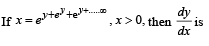Detailed Solution for Test: JEE Main 35 Year PYQs- Differentiation - Question 5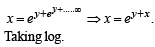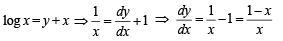Test: JEE Main 35 Year PYQs- Differentiation - Question 6

The value of a for which the sum of the squares of the roots of the equation x2 – (a – 2) x – a – 1 = 0 assume the least value is

Detailed Solution for Test: JEE Main 35 Year PYQs- Differentiation - Question 6

x2 - (a - 2) x -a-1 = 0
⇒ α + b = a- 2 ; α β = -(a+ 1)
α2 + β2 = (α + β)2 - 2αβ = a2 - 2a +6 = (a -1)2+5
For min. value of α2 + β2 where α is an integer
⇒ a = 1.

Test: JEE Main 35 Year PYQs- Differentiation - Question 7

If the roots of th e equation x2 – bx + c = 0 be two consecutive integers, then b2 – 4c equals

Detailed Solution for Test: JEE Main 35 Year PYQs- Differentiation - Question 7

Let α , α + 1 be roots
Then α + α + 1 = b = sum of roots α (α + 1) = c
= product of roots
∴ b2 - 4c = (2a + 1)2 - 4a(a + 1) = 1

Test: JEE Main 35 Year PYQs- Differentiation - Question 8

Let f : R → R be a differentiable function having f (2) = 6,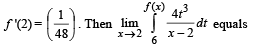Detailed Solution for Test: JEE Main 35 Year PYQs- Differentiation - Question 8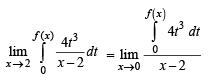Applying L Hospital rule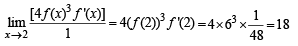Test: JEE Main 35 Year PYQs- Differentiation - Question 9

The set of points where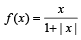is differentiable is

Detailed Solution for Test: JEE Main 35 Year PYQs- Differentiation - Question 9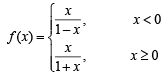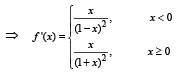∴ f '(x) exist at everywhere.

Test: JEE Main 35 Year PYQs- Differentiation - Question 10

If xm. yn = (x+y) m+n , then dy/dx is

Detailed Solution for Test: JEE Main 35 Year PYQs- Differentiation - Question 10

xm.yn = ( x+y) m+n
⇒ m/nx + n/ny = (m + n)ln(x + y)
Differentiating both sides.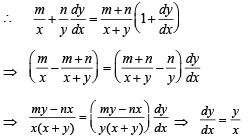Test: JEE Main 35 Year PYQs- Differentiation - Question 11

Let y be an implicit function of x defined by  x2x – 2xx cot y – 1= 0. Then y'(1) equals

Detailed Solution for Test: JEE Main 35 Year PYQs- Differentiation - Question 11

x2x – 2xx cot y – 1 = 0
⇒ 2 cot y = xx –  x – x ⇒ 2 cot y =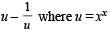Differentiating both sides with respect to x, we get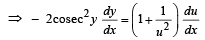where u = xx ⇒ log u = x log x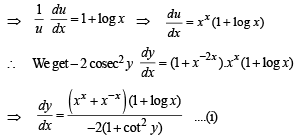Now when x  = 1, x2x – 2xx cot y – 1 = 0, gives
1 – 2 cot y – 1 = 0 ⇒ cot y = 0
∴ From equation (i), at x = 1 and cot y = 0, we get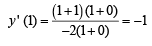Test: JEE Main 35 Year PYQs- Differentiation - Question 12

Let f : (–1, 1) → R be a differentiable function with f(0) = – 1 and f '(0) = 1. Let g(x) = [f (2f (x) + 2)]2. Then g'(0) =

Detailed Solution for Test: JEE Main 35 Year PYQs- Differentiation - Question 12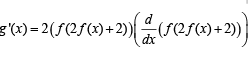= 2 f (2 f (x) + 2) f '(2 f (x))+ 2).(2 f '(x))
⇒ g '(0) = 2 f (2 f (0) + 2). f '( 2 f (0) + 2).2 f '(0)= 4 f (0)(f '(0))2
= 4(–1)(1)2 = – 4

Test: JEE Main 35 Year PYQs- Differentiation - Question 13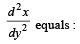Detailed Solution for Test: JEE Main 35 Year PYQs- Differentiation - Question 13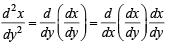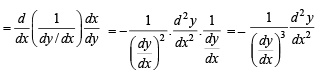Test: JEE Main 35 Year PYQs- Differentiation - Question 14

If y = sec(tan–1x), then dy/dx at x = 1 is equal to :

Detailed Solution for Test: JEE Main 35 Year PYQs- Differentiation - Question 14

Let y = sec(tan–1 x) and tan–1 x = θ.
⇒ x = tan θ

Thus, we have y = sec θ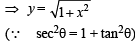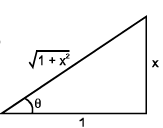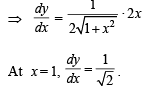Test: JEE Main 35 Year PYQs- Differentiation - Question 15

If g is the inverse of a function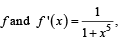then g ' (x) is equal to:

Detailed Solution for Test: JEE Main 35 Year PYQs- Differentiation - Question 15

Since f (x) and g(x) are inverse of each other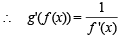⇒ g '(f (x)) =1+x5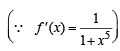Here x = g(y)

∴ g'(y) = 1+ {g (y)}5
⇒ g'( x) = 1+ {g (x)}5

Test: JEE Main 35 Year PYQs- Differentiation - Question 16

If x = –1 and x = 2 are extreme points of f (x) = a log |x| + βx2+x then

Detailed Solution for Test: JEE Main 35 Year PYQs- Differentiation - Question 16

Let  f (x) = α log | x | + βx2 + x Differentiating both sides,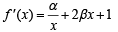Since x = –1 and x = 2 are extreme points therefore f ' (x) =0 at these points.
Put x  = –1 and x = 2 in f '(x) , we get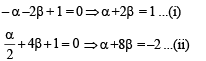On solving (i) and (ii), we get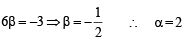## Mathematics (Maths) for JEE Main & Advanced

129 videos|359 docs|306 tests
Information about Test: JEE Main 35 Year PYQs- Differentiation Page
In this test you can find the Exam questions for Test: JEE Main 35 Year PYQs- Differentiation solved & explained in the simplest way possible. Besides giving Questions and answers for Test: JEE Main 35 Year PYQs- Differentiation, EduRev gives you an ample number of Online tests for practice

## Mathematics (Maths) for JEE Main & Advanced

129 videos|359 docs|306 tests(Scan QR code)